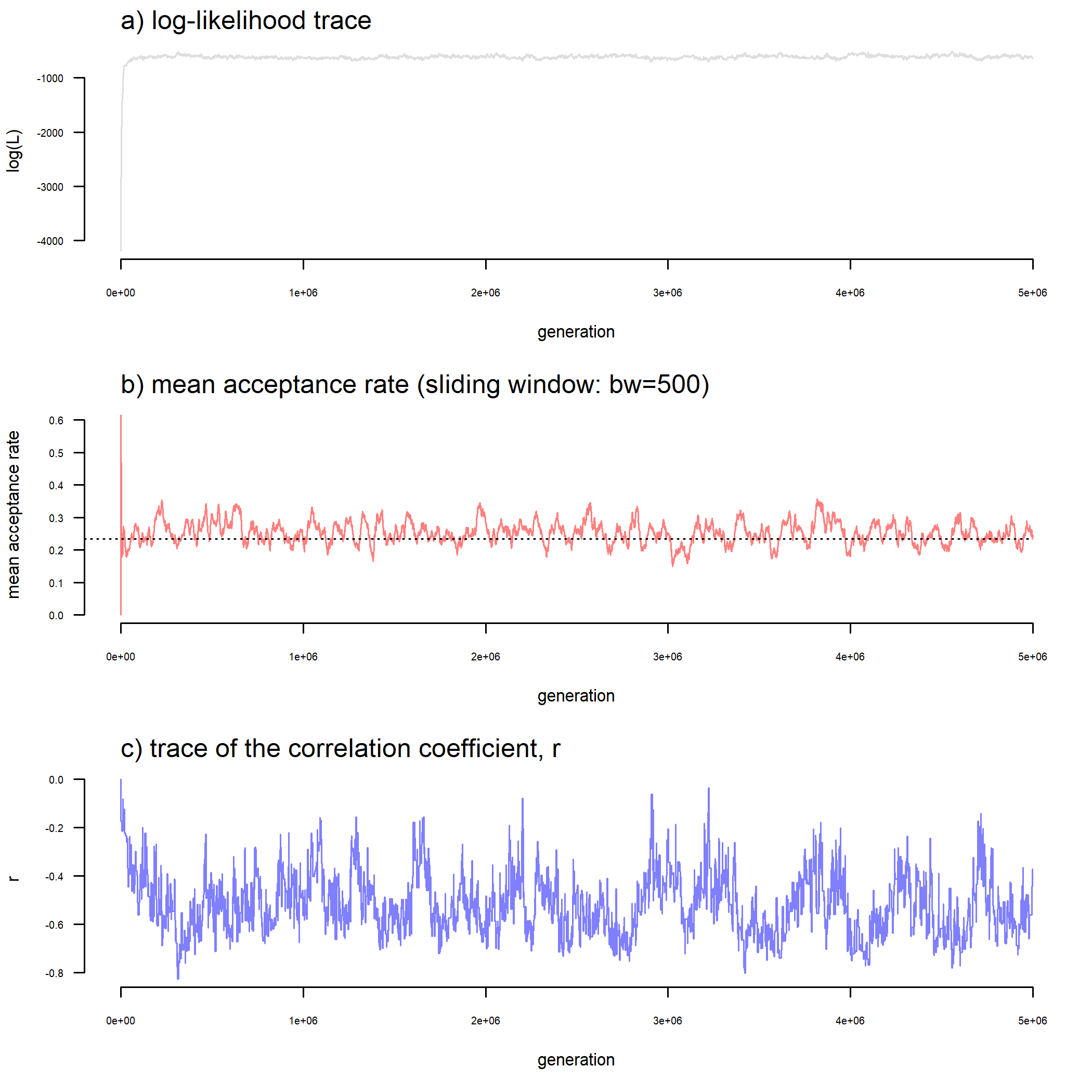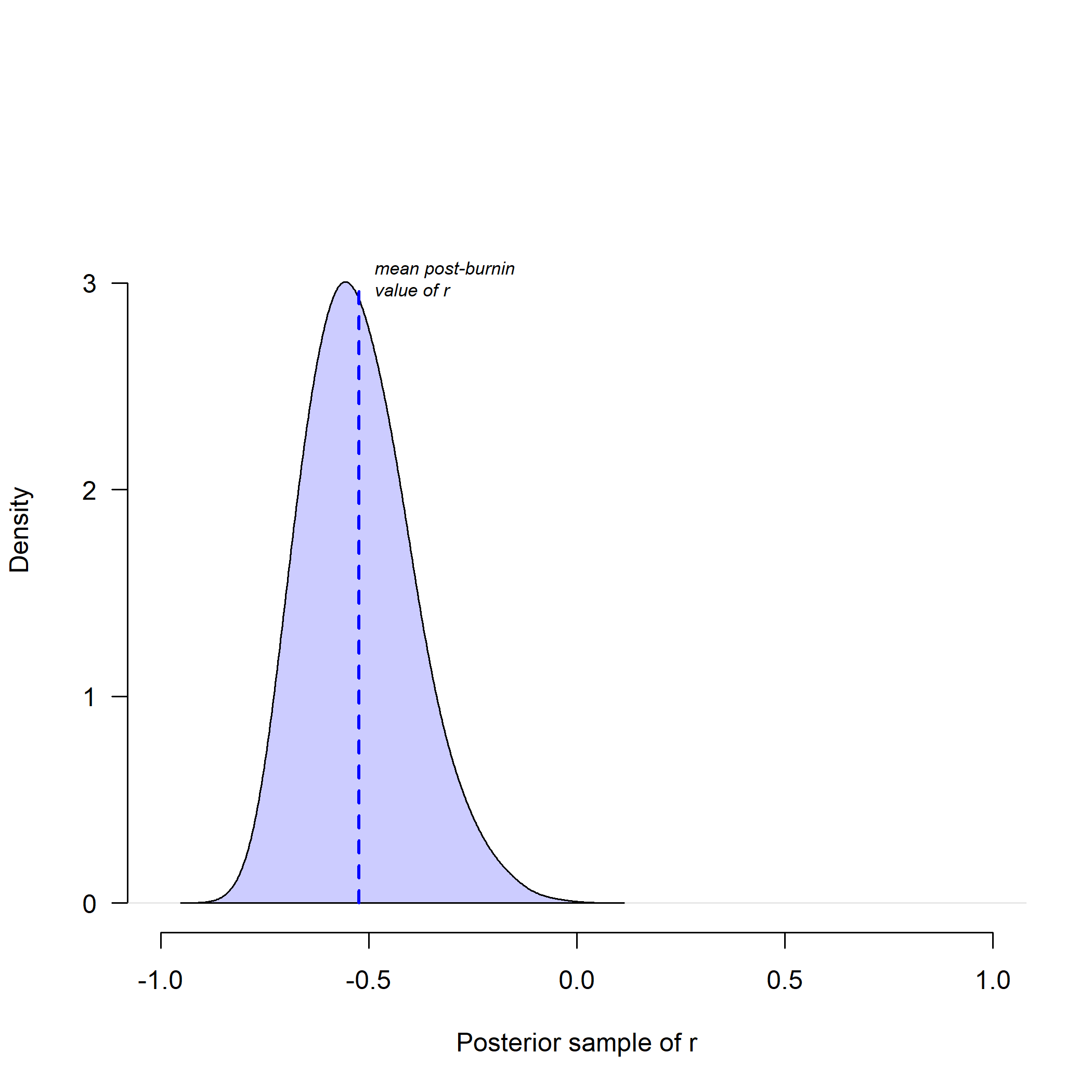## Monday, August 29, 2022

### On the behavior of `threshBayes` for estimating the evolutionary correlation between discrete traits under the threshold model

A friend & phytools user recently contacted me with the following observation:

“A colleague… is trying to use the `threshBayes` function to obtain correlations between discrete traits and unfortunately he is having some weird results. He sent me this minimal example (attached) and I also got the same issues, namely, an incorrect estimate of the correlation parameter and a wide posterior distribution that overlaps with 0. I increased sample sizes to 100 and got the same issue. Any ideas to why that is the case?”

For context, the minimal example involved a simulated evolutionary correlation of the liabilities of 0.5 and a simulated tree size of 10. As note above, the sender also tried an N 10 × larger without much better success.

Remember, the threshold model is one in which the trait value we see is discrete, but it is underlain by an unobserved continuous character, called liability. Whenever liability passes a certain threshold, the discrete character changes in state!

Actually, thresholding a continuous trait in this way throws out so much information, that I'm not all that surprised that both of these minimal examples tended to produce posterior densities that broadly overlapped with zero correlation between the two traits.

What I show below is the same minimal example send to me by the user, but merely updated such that the simulated tree contained 250 tips, and the generating correlation between the liabilities for our two threshold traits was -0.75 instead of 0.5. (I increased the magnitude to make the correlation a bit easier to detect; however, I also made it negative just to show that we should be able to correctly identify the sign of our correlation without excessive difficulty.)

First, let's simulate our tree & trait. This uses the exact code of the minimal example provided by my colleague, but updated with the larger value of N, as mentioned above, and magnitudinally greater evolutionary correlation between the liabilities for the two discrete traits.

``````## set seed for reproducibility
set.seed(0)
library(phytools)
## set simulation conditions
true.r<--0.75
N<-250
## create correlation matrix
cor_real<-rbind(c(1,true.r),c(true.r,1))
## simulate tree
tree<-pbtree(n=N)
tree
``````
``````##
## Phylogenetic tree with 250 tips and 249 internal nodes.
##
## Tip labels:
##   t23, t24, t12, t16, t96, t175, ...
##
## Rooted; includes branch lengths.
``````
``````## simulate liabilities
liabilities<-sim.corrs(tree,as.matrix(cor_real))
## zero center them (just to maximize power)
liabilities<-liabilities-matrix(rep(colMeans(liabilities),N),
N,2,byrow=TRUE)
## threshold to a binary character
foo<-function(...){
x<-threshState(...)
setNames(as.numeric(x),names(x))
}
X<-apply(liabilities,2,foo,thresholds=setNames(c(0,Inf),0:1))
colnames(X)<-c("x1","x2")
``````
``````##      x1 x2
## t23   0  1
## t24   0  0
## t12   1  0
## t16   1  0
## t96   1  0
## t175  1  0
``````
``````mcmc<-threshBayes(tree,X,types=c("disc","disc"),ngen=5e6,plot=FALSE,
control=list(print.interval=1e5))
``````
``````## Starting MCMC....
## generation: 100000; mean acceptance rate: 0.12
## generation: 200000; mean acceptance rate: 0.31
## generation: 300000; mean acceptance rate: 0.21
## generation: 400000; mean acceptance rate: 0.19
## generation: 500000; mean acceptance rate: 0.51
## generation: 600000; mean acceptance rate: 0.25
## generation: 700000; mean acceptance rate: 0.3
## generation: 800000; mean acceptance rate: 0.33
## generation: 900000; mean acceptance rate: 0.18
## generation: 1000000; mean acceptance rate: 0.13
## generation: 1100000; mean acceptance rate: 0.21
## generation: 1200000; mean acceptance rate: 0.25
## generation: 1300000; mean acceptance rate: 0.21
## generation: 1400000; mean acceptance rate: 0.27
## generation: 1500000; mean acceptance rate: 0.34
## generation: 1600000; mean acceptance rate: 0.23
## generation: 1700000; mean acceptance rate: 0.38
## generation: 1800000; mean acceptance rate: 0.16
## generation: 1900000; mean acceptance rate: 0.29
## generation: 2000000; mean acceptance rate: 0.3
## generation: 2100000; mean acceptance rate: 0.18
## generation: 2200000; mean acceptance rate: 0.31
## generation: 2300000; mean acceptance rate: 0.18
## generation: 2400000; mean acceptance rate: 0.31
## generation: 2500000; mean acceptance rate: 0.29
## generation: 2600000; mean acceptance rate: 0.33
## generation: 2700000; mean acceptance rate: 0.33
## generation: 2800000; mean acceptance rate: 0.27
## generation: 2900000; mean acceptance rate: 0.23
## generation: 3000000; mean acceptance rate: 0.28
## generation: 3100000; mean acceptance rate: 0.18
## generation: 3200000; mean acceptance rate: 0.4
## generation: 3300000; mean acceptance rate: 0.29
## generation: 3400000; mean acceptance rate: 0.16
## generation: 3500000; mean acceptance rate: 0.15
## generation: 3600000; mean acceptance rate: 0.26
## generation: 3700000; mean acceptance rate: 0.3
## generation: 3800000; mean acceptance rate: 0.25
## generation: 3900000; mean acceptance rate: 0.3
## generation: 4000000; mean acceptance rate: 0.19
## generation: 4100000; mean acceptance rate: 0.22
## generation: 4200000; mean acceptance rate: 0.39
## generation: 4300000; mean acceptance rate: 0.23
## generation: 4400000; mean acceptance rate: 0.18
## generation: 4500000; mean acceptance rate: 0.15
## generation: 4600000; mean acceptance rate: 0.29
## generation: 4700000; mean acceptance rate: 0.18
## generation: 4800000; mean acceptance rate: 0.2
## generation: 4900000; mean acceptance rate: 0.09
## generation: 5000000; mean acceptance rate: 0.3
## Done MCMC.
``````
``````mcmc
``````
``````##
## Object of class "threshBayes" consisting of a matrix (L) of
## sampled liabilities for the tips of the tree & a second matrix
## (par) with the sample model parameters & correlation.
##
## Mean correlation (r) from the posterior sample is: -0.51999.
##
## Ordination of discrete traits:
##
##  Trait 1: 0 <-> 1
##  Trait 2: 0 <-> 1
``````

Finally, we can plot our results. First, a summary of the MCMC.

``````par(cex.axis=0.6)
plot(mcmc)
``````Then, our posterior density for the correlation coefficient, r.

``````par(las=1)
plot(density(mcmc))
``````What we should see is that although the correlation coefficient tends to be biased towards zero (this is unexpected – it can't take values outside [-1,1]), when we have lots of power, our high probability density interval can easily exclude zero.

As a general rule, it's reasonable to suppose that the mean of the posterior sample from MCMC may be an asymptotically unbiased estimator of the true correlation of the liabilities; however, the long tail of the posterior distribution will always tend point towards zero, just as we see here, and how quickly the asymptote is approached should depend strongly not only on the size of our tree, but also the relative frequency of each state for each trait (greater evenness will increase power).

That's it.